Math Concepts

# Natural numbers: Concepts, Properties and Examples

0

Table of Contents

 1 Introduction to Natural Numbers 2 What are Natural Numbers? 3 Set of Natural Numbers and Introduction for Whole Numbers and Integers 4 Properties of Natural Numbers with Examples 5 Read More Here 6 Solved Examples 7 FAQs

12th December 2020

## Introduction to Natural Numbers

This article will help you to learn more about natural numbers concerning their definition, their comparison with whole numbers, their representation in the number line, and their properties.

Numbers that are used to count are called Natural numbers. The natural numbers are the numbers used for counting for example - there are ten coins in the purse or order for example - Delhi is the third-largest city in the country in terms of population.Also Read:

### Natural numbers: Concepts, Properties, and Examples -PDF

In mathematics, numbers colloquially used for counting are "cardinal numbers", and words used for ordering are "ordinal numbers".  Here is a downloadable PDF to explore more.

 📥 Natural numbers: Concepts, Properties, and Examples -PDF Download

## What are Natural Numbers?

In mathematics, numbers colloquially used for counting are "cardinal numbers", and words used for ordering are "ordinal numbers". For this purpose, if any number is used, it is called a natural number.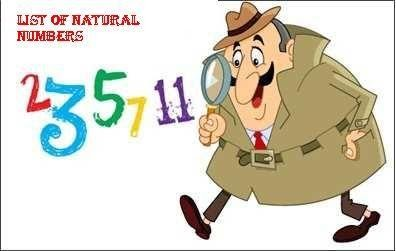Any number which can be made from single or the successive repetition of the following digits is called a natural number.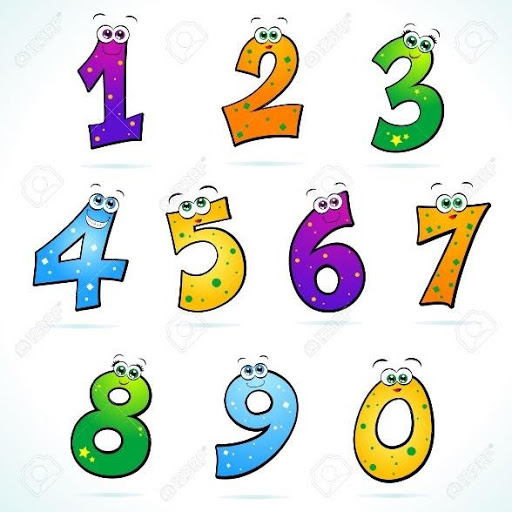Natural numbers do not include zero $$(0)$$. In fact, \begin{align}1,2,3,4,5,6,7,8,9….,\end{align} till infinity are all called counting numbers.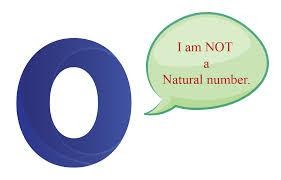Natural numbers exclude zero, fractions, decimals and negative numbers. The set of natural numbers is often denoted by the symbol.The natural numbers are a basis from which many other number sets may be built by extension: the whole numbers, the integers, the rational numbers, the real numbers and the complex numbers. The natural numbers canonically are embedded in all these number systems.

## Set of Natural Numbers and Introduction for Whole Numbers and Integers

Natural numbers are the part of the number system that include all positive integers from $$1$$ uptill infinity. They are also used for counting purpose.
Whole numbers include all the natural numbers along with the number $$0$$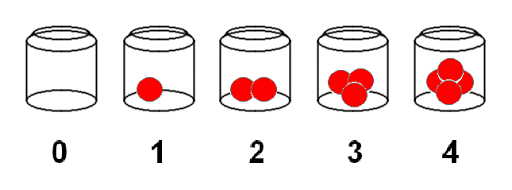In other words, all natural numbers can be whole numbers, but all whole numbers are not natural numbers. For example zero $$(0)$$ is a whole number but not a natural number.

Set of Natural Numbers \begin{align} = 1,2,3,4,5,6,7,8,9,.....\end{align}

Set of Whole Numbers \begin{align}= {0, 1, 2, 3, 4, 5, 6, 7, 8, 9,….}\end{align}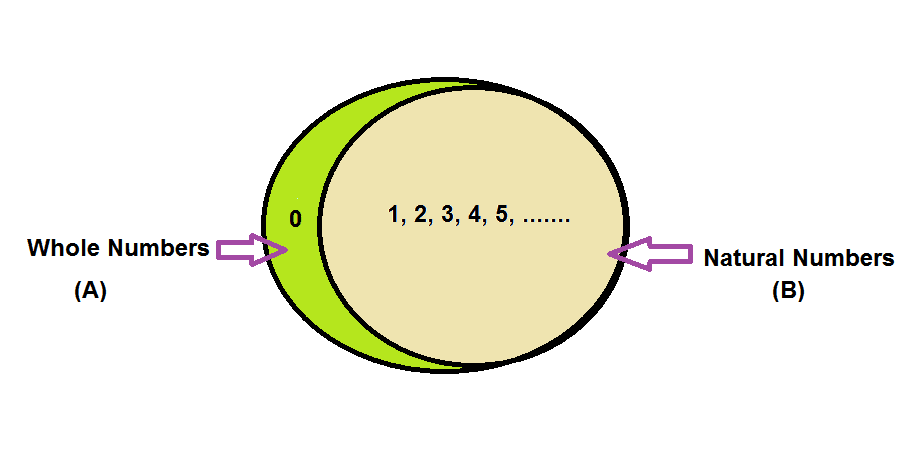The above representation of sets shows two regions natural numbers and whole numbers .Thus a whole number is “a number system consisting of all the natural number including 0.”

## Properties of Natural Numbers with Examples

### Closure Property

Natural numbers are closed under addition and multiplication.

This means when two or more natural numbers are added or multiplied it always results in a natural number. In the case of subtraction and division, natural numbers do not follow closure property, which means subtracting or dividing two natural numbers may not necessarily result in a natural number.

• Addition:\begin{align}4 + 2 = 6, 23 + 44 = 67,\end{align} etc. In each of these cases, the resulting number is a natural number.
• Multiplication:\begin{align}2 × 3 = 6, 25 × 4 = 100\end{align}, etc. In this case also, the resultant is a natural number.
• Subtraction: \begin{align}9 – 1 = 8, 4 \,– 7 = –3,\end{align} etc. In this case, we see that the result may or may not be a natural number.
• Division:\begin{align}20 ÷ 4 = 5, 10 ÷ 3 = 3.33\end{align}, etc. This case also shows that the resultant number may or may not be a natural number.

### Commutative Property

Addition and multiplication of natural numbers follow the commutative property.

\begin{align}a + b + = b + a\,\,\text{and}\,a\, \times b = b \times a\end{align}

Whereas, subtraction and division of natural numbers do not follow the commutative property.

\begin{align}a - b \ne b - a\,\,\text{and}\,\,a \div b \ne b \div a\end{align}

### Associative Property

The associative property holds true in case of addition and multiplication of natural numbers

For addition               \begin{align}a + \left( {b + c} \right) = \left( {a + b} \right) + c\end{align}

For multiplication        \begin{align}a \times (b \times c) = (a \times b) \times c\end{align}.

 For example

For addition               \begin{align}30 + (25 + 11) = 66\,\text{and}\,(30 + 25) + 11 = 66\end{align}

For multiplication       \begin{align}3 \times (15 \times 1) = 45\,\text{and}\,(3 \times 15) \times 1 = 45\end{align}

On the other hand, the associative property does not hold true for subtraction and division of natural numbers.

### Subtraction:

\begin{align}a – ( b – c ) ≠ ( a – b ) – c \end{align}

\begin{align}48 - (15 - 11) = 44\,\,\text{and}\,\,(48 - 15) - 11 = 22\end{align}

### Division:

\begin{align}a \div (b \div c) \ne (a \div b) \div c\end{align}

\begin{align}2 \div (3 \div 6) = 4\,\,\text{and}\,\,(2 \div 3) \div 6 = 0.11\end{align}

### Distributive Property

Multiplication of natural numbers is distributive over addition

\begin{align}a \times (b + c) = a \times b + a \times c\end{align}

Multiplication of natural numbers is distributive over subtraction also.

\begin{align}a \times (b - c) = a \times b - a \times c\end{align}

\begin{align} &5 \times (7 + 3) = 5 \times 10 = 50\,\text{and}\,5 \times 7 + 5 \times 3 = 35 + 15 = 50\\ &9 \times (54 - 45) = 81\,\,\text{and}\,\,9 \times 54 - 9 \times 45 = 486 - 405 = 81 \end{align}

## Read More Here:

Under the study of natural numbers you will study about various other related terms such as odd/even numbers, prime/composite numbers, factors/multiples, HCF/LCM, rounding off/estimation, square/square roots etc.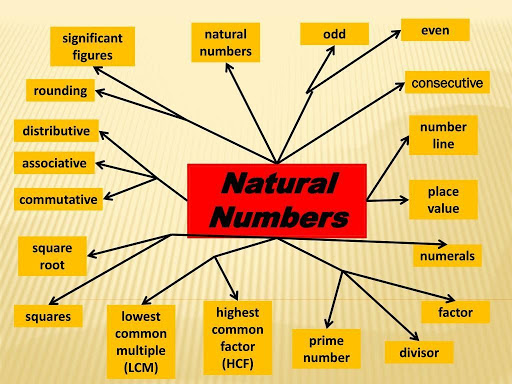## Solved Examples

 Example 1

From the following list of numbers find the natural numbers:

\begin{align} -20, 105, 1535, 63.99, 5/2, 69, −78, 0, −2, −3/2\end{align}

Solution:

 From the above list Natural numbers are $$105, 1535$$ and$$69$$.

 Example 2

Write the first $$10$$ natural numbers?

Solution:

 The first $$10$$ natural numbers are \begin{align}1, 2, 3, 4, 5, 6, 7, 8, 9, 10.\end{align}
 Example 3

Is the number $$0$$ a natural number?

Solution:

 $$0$$ is not a natural number.

 Example 4

What is the sum of first $$10$$ natural numbers?

Solution:

 You can do it by adding number normally. But we will learn it by a simpler way.

Add the numbers as follows:-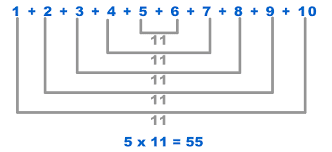## What are Natural Numbers?

Natural numbers are the counting numbers which start from $$1$$ and end at infinity.

\begin{align}1,2,3,4,5,6,7,8,9,10,……,∞.\end{align}

## What are the first twelve Natural Numbers?

The first twelve natural numbers are: \begin{align}1,2,3,4,5,6,7,8,9,10,11,12.\end{align}

## Is $$0$$ a Natural Number?

Zero is not a natural number. Although zero is called a whole number.

## Give some examples of Natural numbers?

The examples of natural numbers are \begin{align}5, 17, 21, 34, 49, 101,\end{align} etc.

## What is the difference between Natural numbers and Whole numbers?

Natural numbers include only positive integers and starts from $$1$$ till infinity. Whereas whole numbers are the combination of zero and natural numbers, as it starts from $$0$$ and ends at infinity.

I hope this article has helped you to get an idea about natural numbers and their properties. We would like to hear from you in case of any query.

## External References

Related Articles
GIVE YOUR CHILD THE CUEMATH EDGE
Access Personalised Math learning through interactive worksheets, gamified concepts and grade-wise courses
Learn More About Cuemath